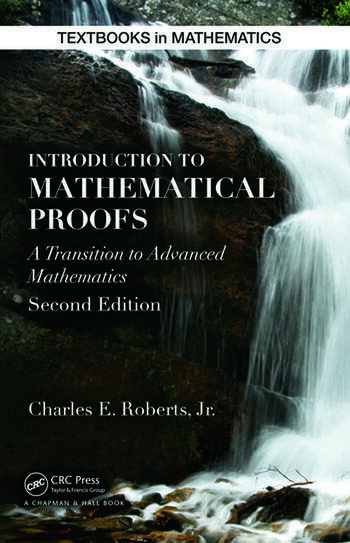Introduction to Mathematical Proofs

2nd Edition

Charles Roberts

Chapman and Hall/CRC
Published December 17, 2014
Textbook - 414 Pages - 51 B/W Illustrations
ISBN 9781482246872 - CAT# K23611
Series: Textbooks in Mathematics

For Instructors Request Inspection Copy

was \$110.00

USD\$88.00

SAVE ~\$22.00

FREE Standard Shipping!

Preview

Summary

Introduction to Mathematical Proofs helps students develop the necessary skills to write clear, correct, and concise proofs.

Unlike similar textbooks, this one begins with logic since it is the underlying language of mathematics and the basis of reasoned arguments. The text then discusses deductive mathematical systems and the systems of natural numbers, integers, rational numbers, and real numbers.

It also covers elementary topics in set theory, explores various properties of relations and functions, and proves several theorems using induction. The final chapters introduce the concept of cardinalities of sets and the concepts and proofs of real analysis and group theory. In the appendix, the author includes some basic guidelines to follow when writing proofs.

This new edition includes more than 125 new exercises in sections titled More Challenging Exercises. Also, numerous examples illustrate in detail how to write proofs and show how to solve problems. These examples can serve as models for students to emulate when solving exercises.

Several biographical sketches and historical comments have been included to enrich and enliven the text. Written in a conversational style, yet maintaining the proper level of mathematical rigor, this accessible book teaches students to reason logically, read proofs critically, and write valid mathematical proofs. It prepares them to succeed in more advanced mathematics courses, such as abstract algebra and analysis.

Instructors

We provide complimentary e-inspection copies of primary textbooks to instructors considering our books for course adoption.